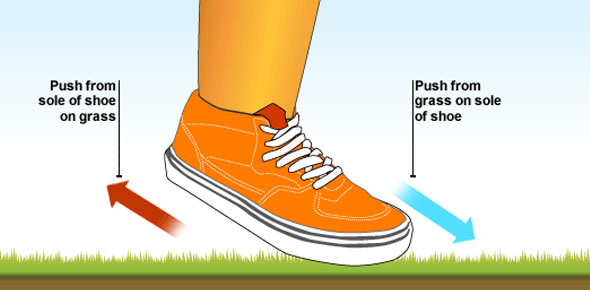# Science Quiz On Motion

8 Questions | Total Attempts: 1237Settings• 1.
The tendancy of an object resists a charge in motion
• A.

Inerta

• B.

Machine

• C.

Work

• 2.
A  device that changes the direction  or the amount of effort needed to do work.
• A.

Acceleration

• B.

Force

• C.

Machine

• 3.
the speed and direction of an objects motion
• A.

Acceleration

• B.

Velocity

• C.

Inerta

• 4.
A push or pull that acts on an object
• A.

Force

• B.

Velcity

• C.

Machine

• 5.
Energy used when a force moves an object
• A.

Movement

• B.

Force

• C.

Work

• 6.
The rate at which work is done
• A.

Power

• B.

Force

• C.

Speed

• 7.
A state at which the net force is 0.
• A.

Power

• B.

Velcity

• C.

Equilibrium

• 8.
the rate at which the velcoity of an object changes over time
• A.

Acceleration

• B.

Average speed

• C.

Velocity

Related TopicsBack to top